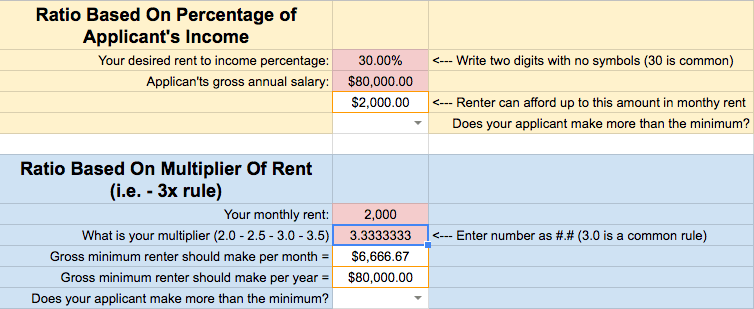# Rent To Income Ratio Calculator – How Much Rent Can Your Tenant Really Afford?

Last Updated on

## Calculating Percentage of Income for Rent Calculator (rent to income ratio)

By using a rent to income ratio standard you can get a general sense of a tenant applicant’s ability to pay rent.

## Ratio Based On Percentage of Applicant’s Income

In this calculation, the final output is the maximum rent that someone can afford based on their salary. A 30% rent to income ratio is very common.

In the next example, we will start with the rent amount and figure out the minimum salary based on that figure.

## Calculating Rent to Income Ratio as a Multiplier of Income

We offer a service called SmartMove which uses a ratio based on the multiplier below:

## Ratio Based On Multiplier Of Rent

The calculators above are a handy tool for quickly figuring things out.

A rent-to-income ratio (sometimes referred to as “income to rent ratio”) is a criteria set up by the landlord for their rental property. This standard sets a threshold of gross income that must be met in order to be considered for the rental property.

If you like spreadsheets and Google Drive you’ll like this next resource…

## Google Sheet For Rent To Income Ratio Calculator

### Easy Mental Math For Rent To Income Ratio

If you’re doing some mental math, simply take an applicant’s gross annual salary, and divide it by 40.

The resulting number will represent 30% of their gross monthly salary.

In other words, the calculation represents the most a tenant can afford to pay for rent based on a 30% rent to income ratio.

### Examples of This Ratio In Use

1. If a tenant makes \$80,000 in gross annual income, you would divide this by 40.
2. The resulting number of \$2,000 is the most that tenant can afford for monthly rent under a 30% ratio.

A longer way of getting to this number is by taking:

1. \$80,000 and dividing it by 12
2. The resulting number of \$6,666.66 represents their gross monthly salary
3. Multiply \$6,666.66 by 30% to get \$2,000

### What Does A 30% Rule Equate To In A Rent To Income Multiplier

A 30% rule is the same as saying a 3.33 (repeating) multiplier ratio.

Here’s a screenshot of those numbers matching up (look at the highlighted box of 3.3333333).

###This is important to understand because if you use a 30% rule than you’re being more selective than someone who uses a 3.0x rule.

### How To Apply This Information

First things first, you should set a proper rental price for your rental.

Once you know what you want to charge monthly, you can easily figure out what annual salary a tenant would need in order to meet your rent to income ratio.

For instance, if you want \$1,200 in monthly rent. You can multiply that number by 40 to get a minimum gross annual salary. In this example it would be \$48,000.

### Things To Know About Income Requirements

You can use rent to income ratio as a legal screening tactic for your rentals.

However, be aware that there are a lot of factors that can alter a persons annual income. Things such as SSI or garnishments are a couple of examples.

You can check out this HUD article to see what income is included or excluded in rent determinations.

[/vc_column_text][/vc_column][/vc_row]